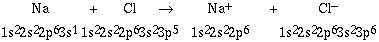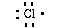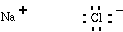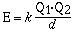### To understand the nature of the ions formed and the basis for the ionic bond we can write the respective electron configurations.### This indicates sodium has one electron in its outer most shell. Chlorine has seven valence electrons in its outer most shell (1s22s22p63s23p5). We can write a Lewis structure for chlorine as;### The new Lewis symbols are;### Another example can be given to examplify this behavior. We'll consider the reaction between a magnesium atom and an oxygen atom.### The charges and sizes of the ions are the critical factors which determine the magnitude of the lattice energy. The lattice energy is related to the potential energy of two interacting charges which is given in the mathematical equation;